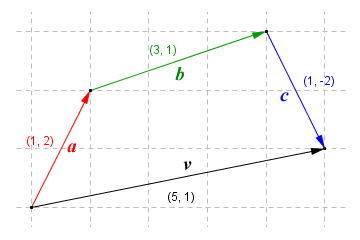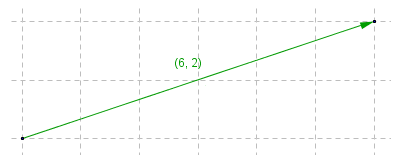# Scalars and Vectors

The physical sciences are by their very nature highly mathematical. Scientists are always asking questions that can only be satisfactorily answered by making calculations or applying formulae to produce a meaningful number or set of results. The calculations made by scientists naturally involve quantities of various kinds. These quantities fall into two basic types - scalar quantities and vector quantities. We will deal with scalar quantities first, since they are relatively straightforward. A scalar quantity is a quantity of something that has magnitude (i.e. size) but not direction. Examples of scalar quantities include length, mass, time, and amount of substance. You may have already realised that each of these examples is one of the base quantities defined in the International System of Units.

Each of the base quantities has a single dimension. We can talk about length, for example, without having to think about a particular direction. Think about questions such as "How long is a piece of string?" or "How far is it from here to the Moon?" These questions have answers that can be expressed as a single number, followed by the appropriate unit symbol. The length of a piece of string might be expressed in inches or centimeters. The distance from the Earth to the Moon would be expressed in kilometres or miles (the average distance is in fact 384 403 kilometres or 238 857 miles). While we're on the subject, the Moon's mass is estimated at 7.34767309 × 1022 kilograms, and it has an estimated age of 4.527 billion years. All of these statistics are, of course, scalar quantities. They have magnitude, but not direction.The moon is approximately 4.527 billion years old
(image credit: Nasa/Bill Ingalls)

The same algebraic operations can be carried out on scalar quantities as on pure numbers. They can be added or multiplied together, and one scalar value can be divided by or subtracted from another scalar value. The normal rules of arithmetic do of course apply to algebraic operations involving scalar quantities. There are however one or two things to be aware of. The first is that, unlike pure numbers, scalar quantities can refer to different types of physical quantity. You can only use operations like addition, subtraction, multiplication and division on scalar quantities of the same type. You can add ten kilograms (10 kg) to five hundred grams (500 g) for example, but you must express both amounts in the same units (either grams or kilos). Adding a quantity of length or time to a quantity of mass, on the other hand, would produce a result that is totally meaningless.

Multiplying or dividing a scalar quantity by a pure number is perfectly legitimate. For example, if we had five (5) identical gold bars, each with a mass of six kilograms (6 kg), we could multiply six kilograms by five to obtain a total mass of thirty kilograms (6 kg × 5 = 30 kg). When multiplying two scalar quantities together, however, we must multiply the units together as well. If we multiple three metres (3 m) by five metres (5 m), for example, we get the result fifteen square metres (15 m2). This is the kind of calculation we would typically make to find the area of some surface (the surface area of a solar panel, for example), so an answer expressed in square metres would make sense.

Quantities that have a directional component are called vector quantities. Quantities such as displacement, velocity and force have direction as well as magnitude, so they are all vector quantities. Most of the vector quantities you will come across in the study of physics will have either two components (vectors that indicate a magnitude and direction in a two-dimensional plane) or three components (vectors that indicate a magnitude and direction in a three-dimensional space). Two-dimensional vectors lie in the same plane, and are therefore said to be co-planar. The components of a vector are usually (though not always) perpendicular (at right-angles) to one another. In a two-dimensional environment, for example, a vector will have an x component and a y component, as shown in the diagram below. The magnitude of a vector is indicated in the diagram by its length.In a two-dimensional plane, a vector has an x component and a y component

From the above diagram, we can see that vectors can be represented graphically using arrows. The head of the arrow points in the direction of the vector, while the length of the arrow represents the vector's magnitude. You might also have realised that the x and y components of a vector are themselves vectors, although essentially one-dimensional vectors. Vector v has component vectors x and y. This means that vector v is the result of adding vectors x and y together. The vector we obtain by adding two vectors together in this way is called the resultant. We can add any number of vectors together, as demonstrated by the vector diagram below. The vectors are simply placed so that the head of the first vector points to the tail of the next vector, and so on. You can arrange the vectors in any order and get the same result.When vectors a, b and c are added together, the resultant is vector v

Vector v in this example is the resultant of adding vectors a, b and c. Note that it has x and y components of five (5) and one (1) respectively. We can add vectors together without having to draw a vector diagram simply by summing their x and y components to find the x and y components of the resultant. This is a far more concise (and often less error-prone) way of adding vectors. One interesting thing to note here is that, unless all of the vectors being added together are pointing in exactly the same direction, the magnitude of the resultant will always be less than the combined magnitude of the component vectors. This makes sense when you think about it, since the shortest distance between two points is always a straight line. In some cases, the resultant may be the zero vector, if both the x components and the y components of the vectors being added together sum to zero.

Subtracting one vector from another is also relatively straightforward. We can represent vector subtraction graphically, as shown below. Here, we subtract vector b from the sum of vectors a and c. We effectively achieve this by reversing the direction of vector b, which as a result is now shown on the diagram as vector -b. Note that when subtracting a vector algebraically, the easiest method is simply to negate the values of the x and y components of the vector to be subtracted, and then just find the sums of the x and y components, as we would do for any other vector addition. We simply add vectors a, -b and c together instead of subtracting vector b from the sum of vectors a and c.Here is the result of adding vectors a, -b and c

Multiplying a vector by a scalar value is a relatively straightforward operation, and produces another vector. Simply multiply both the x and the y components of the original vector by the scalar value to get the x and y components of the new vector. The direction of the new vector will be exactly the same as that of the original vector. It is of course possible to multiply the x and y components of a vector by two different scalar values, which produces a vector that points in a different direction. We can also multiply a vector by a negative number, in which case the resulting vector will point in the opposite direction. A special case of this is where we multiply a vector by minus one (-1). This leaves the magnitude (i.e. length) of the vector unchanged, and simply reverses its direction. Multiplying a vector by any negative value other than minus one will both reverse the direction and change the magnitude of the vector. Let's look at a couple of examples.Here is the result of multiplying the vector (3, 1) by two (2):We now have the vector (6, 2)

Here is the result of multiplying the vector (3, 1) by minus one (-1):Multiplying vector (3, 1) by minus one (-1) gives us vector (-3, -1)

We have already mentioned the magnitude of vectors several times, but how do we actually find the magnitude of a vector if we only have its x and y components? The answer is relatively straightforward. Here once more is the first vector we looked at. This time, the x and y component vectors have been arranged so that they form the legs of a right-angled triangle, for which vector v forms the hypotenuse.Vector v is the hypotenuse of a right-angled triangle

We can find the magnitude of vector v (i.e. the length of the vector) using Pythagoras' theorem. Here is the calculation:

v  =  √(x 2  +  y 2)  =  √(16  +  9)  =  25  =  5

You will come across vectors frequently when studying the physical sciences. Physicists, for example, are interested in the forces acting upon an object that is at rest or in motion. Force is a vector quantity because it has both magnitude and direction. We often need to apply a force to an object in order to achieve some task. Examples might include such diverse activities as lifting a block of concrete into position, towing a trailer or caravan, or placing a communications satellite into orbit. In some cases, the force required to achieve a task must be applied indirectly. We therefore need to be able to resolve forces into their component vectors in order to work out how much force must be applied in a given direction in order to achieve a particular task. The vector arithmetic involved in this kind of calculation is somewhat beyond what we have discussed here. A far more detailed treatment of vectors can be found in the Mathematics section of this website, in the section entitled "Vectors and Vector Arithmetic".